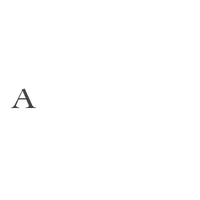Rs. 46,000

Rs. 46,000

Rs. 55,000

Rs. 55,000

Rs. 100,000

Rs. 117,000

Rs. 127,000

Rs. 135,000

Rs. 155,000

Rs. 170,000

Rs. 172,000

Rs. 175,000

Rs. 185,000

Rs. 185,000

Rs. 185,000

Rs. 185,000

Rs. 185,000

Rs. 185,000

Rs. 195,000

Rs. 196,000

Rs. 197,000

Rs. 205,000

Rs. 205,000

Rs. 210,000

Rs. 237,000

Rs. 237,000

Rs. 237,000

Rs. 240,000

Rs. 242,000

Rs. 245,000

Rs. 245,000

Rs. 255,000

Rs. 262,000

Rs. 270,000

Rs. 305,000

Rs. 327,000

Rs. 330,000

Rs. 362,000

Rs. 385,000

Rs. 410,000

Rs. 555,000

Rs. 423,000

Rs. 423,000

Rs. 440,000

Rs. 450,000

Rs. 455,000

Rs. 470,000

Rs. 471,000

Rs. 475,000

Rs. 490,000

Rs. 492,000

Rs. 540,000

Rs. 590,000

Rs. 590,000

Rs. 606,000

Rs. 606,000

Rs. 625,000

Rs. 629,000

Rs. 640,000

Rs. 660,000

Rs. 700,000

Rs. 700,000

Rs. 701,000

Rs. 711,000

Rs. 732,000

Rs. 780,000

Rs. 780,000

Rs. 795,000

Rs. 860,000

Rs. 950,000

Rs. 980,000

Rs. 1,072,000

Rs. 1,267,000

Rs. 1,350,000

Rs. 1,350,000

Rs. 1,394,000

Rs. 1,550,000